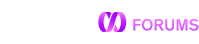# Sorted GROUP_CONCAT

Is it possible to sort the values in a GROUP_CONCAT? There does not appear to be an ORDER BY clause and I tried nesting the sort but it did not seem to work. If not, are there any other ways to achieve this requirement? I found this post on StackOverflow, which suggests using an analytical function such as row number and sorting by that.

This syntax will work in the upcoming 7.0 release:

``````SELECT dept_id,
GROUP_CONCAT (emp_id ORDER BY emp_id SEPARATOR', ')
as "employees ids"
from employee group by dept_id;
``````

But ORDER BY in GROUP_CONCAT is not supported in 6.8. A possible workaround would be to write a UDF, say SORT_GROUP, to parse the result of GROUP_CONCAT and sort it in an array, e.g.

``````SELECT dept_id,
SORT_GROUP(GROUP_CONCAT (emp_id SEPARATOR', '))
as "employees ids"
from employee group by dept_id;
``````

The SPLIT function (https://docs.memsql.com/sql-reference/v6.8/split/) would make it easier to write.

Is there a function to sort an array or does that need to be coded in this function too? I can’t find a documented list of array functions such as LENGTH, although it’s used in examples. (LENGTH is only documented as a string function.)

Also, I’m using 6.7, so the SPLIT function isn’t available either.I came up with this for now.

DELIMITER //
CREATE OR REPLACE FUNCTION TEST(I_STRING LONGTEXT, I_OLD_SEP LONGTEXT, I_NEW_SEP LONGTEXT) RETURNS LONGTEXT AS
DECLARE
V_STRING LONGTEXT;
V_NEXT_SEP INT;
A_SPLIT ARRAY(LONGTEXT);
V_ELEM_COUNT INT=-1;
V_SWAP LONGTEXT;
V_RESULT LONGTEXT;
BEGIN
V_STRING=I_STRING;
V_NEXT_SEP=INSTR(V_STRING,I_OLD_SEP);

WHILE V_NEXT_SEP>0 LOOP
A_SPLIT+=CREATE_ARRAY(1);
V_ELEM_COUNT=V_ELEM_COUNT+1;
A_SPLIT[V_ELEM_COUNT]=LEFT(V_STRING,V_NEXT_SEP-1);
V_STRING=SUBSTR(V_STRING,V_NEXT_SEP+1);
V_NEXT_SEP=INSTR(V_STRING,I_OLD_SEP);
END LOOP;

IF V_STRING IS NOT NULL THEN
V_ELEM_COUNT=V_ELEM_COUNT+1;
A_SPLIT+=CREATE_ARRAY(1);
A_SPLIT[V_ELEM_COUNT]=V_STRING;
END IF;

FOR i IN 0 … LENGTH(A_SPLIT)-2 LOOP
IF A_SPLIT[i]>A_SPLIT[i+1] THEN
V_SWAP=A_SPLIT[i];
A_SPLIT[i]=A_SPLIT[i+1];
A_SPLIT[i+1]=V_SWAP;
END IF;
END LOOP;

FOR i IN 0 … LENGTH(A_SPLIT)-1 LOOP
IF i=0 THEN
V_RESULT=A_SPLIT[i];
ELSE
V_RESULT=CONCAT(V_RESULT,I_NEW_SEP,A_SPLIT[i]);
END IF;
END LOOP;

RETURN V_RESULT;
END //
DELIMITER ;

I made note of your requests to document LENGTH for arrays and to add a built-in ARRAY sort routine.

1 Like

Looks like your sort loop won’t always leave the array fully sorted. May need to add an outer loop or use another approach.

1 Like

Thanks for pointing that out. I wrote that based on some sort pseudo-code I found, and it worked for my first test cases, but I see why that won’t always work.

This seems to work.

DELIMITER //
CREATE OR REPLACE FUNCTION `SORT_LIST`(I_LIST longtext CHARACTER SET utf8 COLLATE utf8_general_ci, I_OLD_SEP longtext CHARACTER SET utf8 COLLATE utf8_general_ci, I_NEW_SEP longtext CHARACTER SET utf8 COLLATE utf8_general_ci) RETURNS longtext AS
DECLARE
V_TEMP LONGTEXT;
A_LIST ARRAY(LONGTEXT);
V_LIST_INDEX INT=-1;
V_NEXT_SEP INT;
V_SORTED_LIST LONGTEXT;

BEGIN
V_TEMP=I_LIST;
V_NEXT_SEP=INSTR(V_TEMP,I_OLD_SEP);

WHILE V_NEXT_SEP>0 LOOP
A_LIST+=CREATE_ARRAY(1);
V_LIST_INDEX=V_LIST_INDEX+1;
A_LIST[V_LIST_INDEX]=LEFT(V_TEMP,V_NEXT_SEP-1);
V_TEMP=SUBSTR(V_TEMP,V_NEXT_SEP+1);
V_NEXT_SEP=INSTR(V_TEMP,I_OLD_SEP);
END LOOP;

IF V_TEMP IS NOT NULL THEN
V_LIST_INDEX=V_LIST_INDEX+1;
A_LIST+=CREATE_ARRAY(1);
A_LIST[V_LIST_INDEX]=V_TEMP;
END IF;

FOR i IN 0 … LENGTH(A_LIST)-1 LOOP
FOR j IN 0 … LENGTH(A_LIST)-i-2 LOOP
IF A_LIST[j]>A_LIST[j+1] THEN
V_TEMP=A_LIST[j];
A_LIST[j]=A_LIST[j+1];
A_LIST[j+1]=V_TEMP;
ELSIF A_LIST[j]=A_LIST[j+1] THEN
A_LIST[j+1]=NULL;
END IF;
END LOOP;
END LOOP;

FOR i IN 0 … LENGTH(A_LIST)-1 LOOP
IF A_LIST[i] IS NOT NULL THEN
IF i=0 THEN
V_SORTED_LIST=A_LIST[i];
ELSE
V_SORTED_LIST=CONCAT(V_SORTED_LIST,I_NEW_SEP,A_LIST[i]);
END IF;
END IF;
END LOOP;

RETURN V_SORTED_LIST;
END//
DELIMITER ;